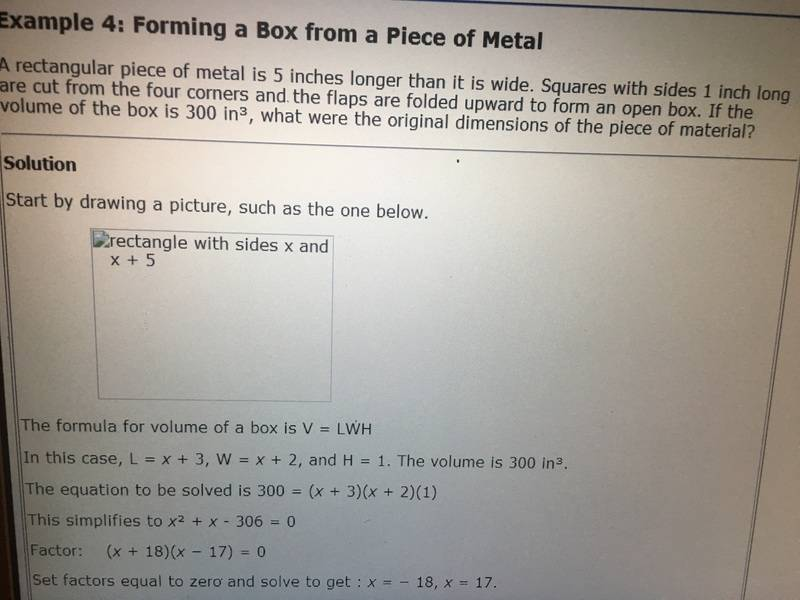# How to solve this quadratic equation?

SunnyBoy123
New user has been reminded to use the Homework Help Template and show their work in the first post
Ok so what am I doing wrong, when i try to put equation 300 = (x+3)(x+2)(1) into Stanford form I get x^2 + 5x - 294 = 0.

http://imgur.com/a/N9E83

Ray Vickson
Homework Helper
Dearly Missed
Ok so what am I doing wrong, when i try to put equation 300 = (x+3)(x+2)(1) into Stanford form I get x^2 + 5x - 294 = 0.

http://imgur.com/a/N9E83

There is no such thing as a "Stanford form", but there can be a "standard form". Anyway, you have re-written the equation correctly.

lewando
Homework Helper
Gold Member
The equation that you have correctly re-written is wrong.

Ray Vickson
Homework Helper
Dearly Missed
The equation that you have correctly re-written is wrong.

This is probably very confusing to the OP. You are literally saying that the equation ##(x+2)(x+3) = 300## is wrong, because that is the equation he re-wrote. In fact, the equation is correct and his re-write of it is also correct; the book's re-write is wrong.

Last edited:
lewando
Homework Helper
Gold Member
The equation that you have correctly re-written is wrong.
Sorry--upon re-read this is unclear. My intent was to encourage him to look upstream a bit more.

SammyS
Staff Emeritus